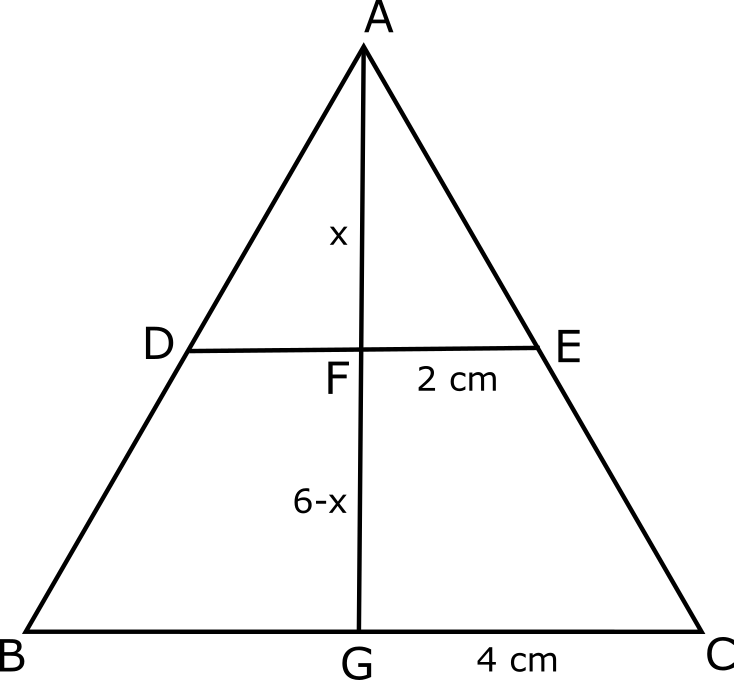# CAT Questions on Cones and Frustum

1
2263

CAT Questions on Cones and Frustum:

Practice important mensuration questions on Cones and Frustum. Important solved problems on Geometry Cones topic based on previous solved question papers.

Question 1: A cylinder and a cone have the same base radius and the same height. The radius of the cylinder is doubled and its height is tripled while the radius of the cone is tripled and its height is doubled. What is the ratio of the new volumes of the cylinder and the cone?

a) 3:1
b) 1:3
c) 1:2
d) 2:1

Question 2: There is a cone of radius 4 cm and height 6 cm standing on its base. Now a portion of the cone from the top is cut such that we now have a smaller cone of radius 2 cm and the frustum of the bigger cone. What is the ratio of the volume of the smaller cone to the volume of the frustum of the cone?

a) 1:14
b) 1:7
c) 3:35
d) 2:27

Question 3: A cone of radius 3 cm and height 4 cm is to be painted from the outside (including the base). If the cost of painting per sq. cm is Rs. 14, then what would be the total cost incurred in painting the cone?

a) Rs. 1056
b) Rs. 2112
c) Rs. 528
d) Rs. 512
e) Rs. 1024

Question 4: A solid sphere is melted and recast into a right circular cone with a base radius equal to the radius of the sphere. What is the ratio of the height and radius of the cone so formed?

a) 4:1
b) 3:1
c) 4:3
d) 3:2

Question 5: Find the altitude to the side BC of the triangle ABC if AB = 10, AC = 10 cm and BC = 16 cm.

a) 6 cm
b) 4 cm
c) 8 cm
d) 5 cm

Solutions for CAT Questions on Cones and Frustum:

Solutions:

Let the initial radius of cylinder and cone be ‘r’ and height be ‘h’
So for cylinder, the new radius is 2r and height is 3h
The volume of the cylinder is $\pi (2r)^{2}*3h = 12 \pi r^{2}h$
The new radius of cone is 3r and its new height is 2h
So the required volume is $\frac{1}{3}\pi(3r)^{2}*2h = 6 \pi r^{2}h$
Hence the required ratio is 2:1

=The volume of a cone with radius ‘r’ and height ‘h’ is $\frac{1}{3}\times\pi\times r^2\times h$.
The volume of a frustum of a cone with radii ‘r’ and ‘R’ and height ‘h’ is $\frac{1}{3}\times\pi\times h\times (R^2+Rr+r^2)$
The cross section of the cone will be as shown in the figure below.ABC is the cross section of the larger cone and ADE is the cross section of the smaller cone.
Let ‘x’ be the height of the smaller cone.
In the figure, triangles AFE and AGC are similar.
So, $\frac{AF}{AG}=\frac{EF}{CG}=>\frac{x}{x+(6-x)}=\frac{2}{4}=>x=3$ cm
Now the volume of the smaller cone is $\frac{1}{3}\times\pi\times 2^2\times 3=4\pi$
The volume of the frustum of the bigger cone is $\frac{1}{3}\times\pi\times 3\times (4^2+4\times2+2^2)=28\pi$
The ratio of volumes is $4\pi:28\pi=1:7$

$l=\sqrt{r^2+h^2}= \sqrt{3^2+4^2}=5$ cm
Total surface area of the cone
= $\pi r^2+\pi rl$
= $\pi \times (9+15)$
= $24\pi$ $cm^2$
Total cost of painting
= $24\times\frac{22}{7}\times14$
= Rs. 1056

Let the radius of the sphere be ‘r’. Now volume of sphere would be equal to the volume of the cone. So
$\frac{4}{3}\pi r^{3} = \frac{1}{3}\pi r^{2}h$
=> $\frac{4}{3}r = \frac{1}{3}h$
=> h/r = 4
Hence the required ratio is 4:1

==>Altitude $AD^2 = 10^2 – 8^2$ = 36\$ ==> AD = 6 cm.